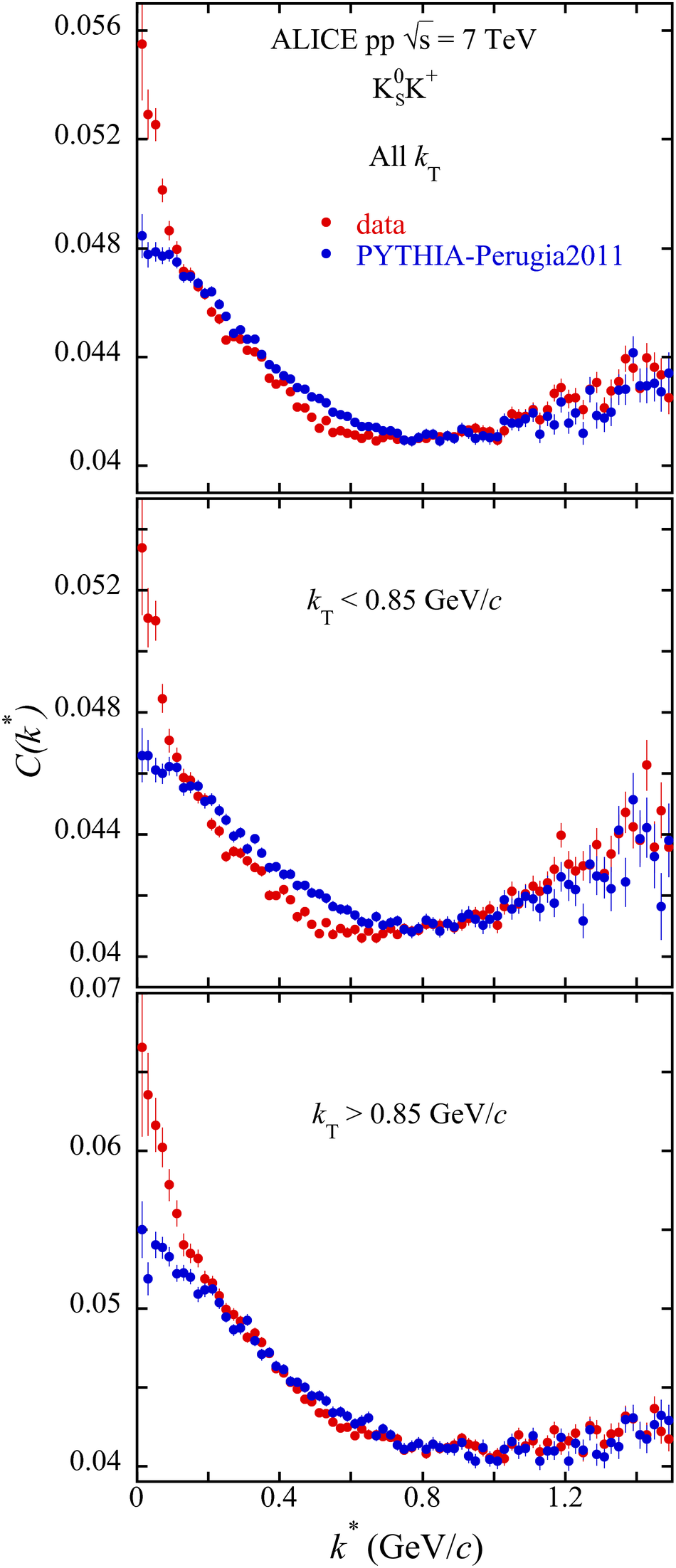# Figure 1

 Raw K$^0_{\rm S}$K$^{\rm +}$ correlation functions for the three $k_T$ bins compared with those from PYTHIA. The error bars are statistical. The scale of $C(k^*)$ is arbitrary. The PYTHIA correlation functions are normalized to the datain the vicinity of $k^*=0.8$ GeV/$c$.